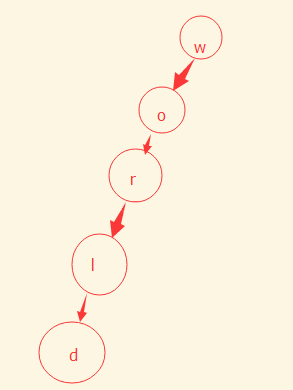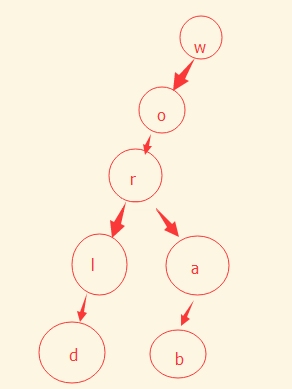PHP字典树(Trie树)定义与实现方法示例

Trie树的概念（百度的解释）：字典树又称单词查找树，Trie树，是一种树形结构，是一种哈希树的变种。典型应用是用于统计，排序和保存大量的字符串（但不仅限于字符串），所以经常被搜索引擎系统用于文本词频统计。它的优点是：利用字符串的公共前缀来减少查询时间，最大限度地减少无谓的字符串比较，查询效率比哈希树高。PHP实现代码：

```<?php
class Node{
public \$value;         // 节点值
public \$is_end = false;    // 是否为结束--是否为某个单词的结束节点
public \$childNode = array();  // 子节点
/* 添加孩子节点--注意：可以不为引用函数，因为PHP对象赋值本身就是引用赋值 */
public function &amp;addChildNode(\$value, \$is_end = false){
\$node = \$this->searchChildNode(\$value);
if(empty(\$node)){
// 不存在节点，添加为子节点
\$node = new Node();
\$node->value = \$value;
\$this->childNode[] = \$node;
}
\$node->is_end = \$is_end;
return \$node;
}
/* 查询子节点 */
public function searchChildNode(\$value){
foreach (\$this->childNode as \$k => \$v) {
if(\$v->value == \$value){
// 存在节点，返回该节点
return \$this->childNode[\$k];
}
}
return false;
}
}
/* 添加字符串 */
\$node = null;
for (\$i=0; \$i < strlen(\$str); \$i++) {
if(\$str[\$i] != ' '){
\$is_end = \$i != (strlen(\$str) - 1) ? false : true;
if(\$i == 0){
}else{
}
}
}
}
/* 获取所有字符串--递归 */
function getChildString(\$node, \$str_array = array(), \$str = ''){
if(\$node->is_end == true){
\$str_array[] = \$str;
}
if(empty(\$node->childNode)){
return \$str_array;
}else{
foreach (\$node->childNode as \$k => \$v) {
\$str_array = getChildString(\$v, \$str_array, \$str . \$v->value);
}
return \$str_array;
}
}
/* 搜索 */
function searchString(\$node, \$str){
for (\$i=0; \$i < strlen(\$str); \$i++) {
if(\$str[\$i] != ' '){
\$node = \$node->searchChildNode(\$str[\$i]);
// print_r(\$node);
if(empty(\$node)){
// 不存在返回空
return false;
}
}
}
return getChildString(\$node);
}
/* 调用测试开始 */
// 添加单词
// 获取所有单词
// 搜索